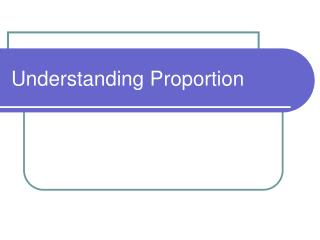DownloadDownload PresentationUnderstanding Proportion

Understanding Proportion

Download PresentationUnderstanding Proportion

- - - - - - - - - - - - - - - - - - - - - - - - - - - E N D - - - - - - - - - - - - - - - - - - - - - - - - - - -
Presentation Transcript

1. Understanding Proportion

2. Ratio • A ratio is the comparison of two numbers by division. • A classroom has 16 boys and 12 girls. • Also written as 16 boys, 16:12 or 16 to 12 12 girls • Generally, ratios are in lowest terms: 16 = 16/4 = 4 12 12/4 3

3. Ratio, continued • Ratios can compare two unlike things: • Joe earned \$40 in five hours • The ratio is 40 dollars or 8 dollars 5 hours 1 hour • When the denominator is one, this is called a unit rate.

4. Ratio, continued Let’s look at a classroom: • Ratios can be part-to-part • 16 boys15 girls • Ratios can be part-to-whole • 16 boys31 students

5. Ratio, continued • If a ratio is part-to-whole, you can divide and find a decimal or a percent. • 16 boys31 students 31/16.00 = .516, or 51.6%are boys

6. Proportion • Proportion is a statement that says two ratios are equal. • In an election, Damon got three votes for each two votes that Shannon got. Damon got 72 votes. How many votes did Shannon get? • Damon 3 = 72 so 3 x 24 = 72 Shannon 2 n 2 x 24 48 n = 48, so Shannon got 48 votes.

7. Proportion, continued • Tires cost two for \$75. How much will four tires cost? • # of tires2 = 4 so 2 x 2 = 4 tires cost 75 n 75 x 2 \$150 n = 150, so four tires cost \$150

8. Proportion, continued • One more way to solve proportions: • 2 = 62 x n = 6 x 82n = 488 n 2 2 n = 24

9. Proportion, continued • Now you try! • Three cans of soup costs \$5. How much will 12 cans cost? • # of cans3 = 123 x 4 = 12 cans cost 5 n 5 x 4 20 dollars n = 20, so 12 cans cost \$20